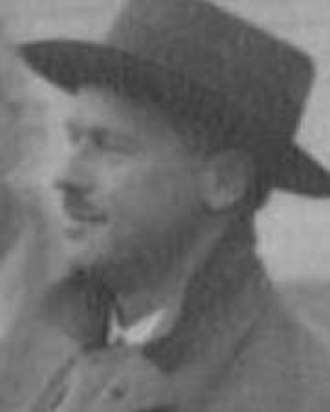A# Bieberbach conjectureLudwig Bieberbach.

The Bieberbach conjecture is a celebrated conjecture made by the German mathematician Ludwig Bieberbach (1886–1982) in 1916, which was finally proved, after many partial results by others, by Louis de Branges of Purdue University in 1984.1 Bierberbach is infamous in the history of mathematics because of his outspoken anti-Semitism during the Nazi era. Following the dismissal of Edmund Landau (1877–1938) from the University of Göttingen, Bierberbach wrote: "This should be seen as a prime example of the fact that representatives of overly different races do not mix as students and teachers... The instincts of the Göttingen students felt that Landau was a type who handled things in an un-German manner."

Bieberbach's conjecture (BC) stemmed from the Riemann conjecture (RC), which makes a claim about any region of a plane that is simply-connected (in other words, any region, however complicated, that doesn't have any holes). RC says there must be some function, or mapping, such that every point in the arbitrary region is associated with one and only one point inside a circle with unit radius. Complex functions are best suited to plane-to-plane mappings and are often easier to work with if they can be represented as a power series. For example, given the complex number z, the function ez can be expressed as the infinite series 1 + z + z2/2! + z3/3! + ... Bieberbach guessed that there is a link between the conditions imposed on a function by RC and the numerical coefficients of the terms in a power series that represents the function. The BC says that if a function gives a one-to-one association between points in the unit circle and points in a simply-connected region of the plane, the coefficients of the power series that represents the function are never larger than the corresponding power. In other words, given that f(z) = a0 + a1z + a2z2 + a3z3 + ... then |an| ≤ n |a1| for each n.

### Reference

1. Branges, L., de "A Proof of the Bieberbach Conjecture." Acta Math., 154: 137-152 (1985).